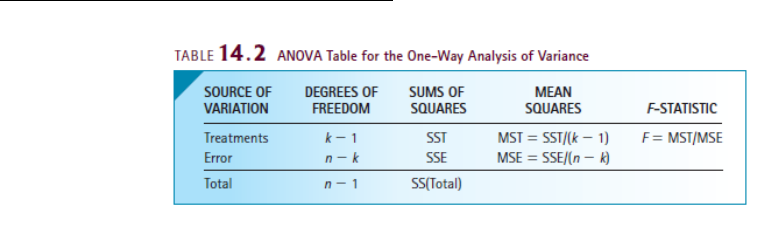Textbook Notes (280,000)
CA (170,000)
York (10,000)
OMIS (50)
Chapter 14

# OMIS 2010 Chapter Notes - Chapter 14: Dependent And Independent Variables, Royal Institute Of Technology, Ssab

Department
Operations Management and Information System
Course Code
OMIS 2010
Professor
Alan Marshall
Chapter
14

This preview shows pages 1-2. to view the full 7 pages of the document.Chapter 14: Analysis of Variance (ANOVA)
-This chapter helps compare two or more populations of interval data
-Determines whether differences exist between populations means
-Analyzes sample variance
One-Way ANOVA
-ANOVA tests to determine whether differences exist between two or more population means
-One way ANOVA: procedure used when samples are independently drawn
oFor each population, we draw independent random samples
o“j” nth sample
o“k” number of populations
o“X” response variable
oUnits measured are called experimental units
oFactor: criterion by which we classify the populations
oEach population called a Factor level
Test Statistic
-If null hypothesis is true, population means would all be equal; H0 is always states that there is no
difference between the means
-H1 always says at least two means differ
-Between treatment variation or SST: measures proximity of sample means to each other
oIf sample means close to each other, all of the sample means would be close to the grand
mean and SST will be small
oIf all means are the same, SST = 0
oIf differences between sample means exist, a large value of SST will be produced
oVariation attributed to differences between treatment means
-Within-treatments variation or SSE: measures amount of variation in the response variable that
is not cause by the treatments
oGroup all sources of variation and call them errors
This variation is measured by sum of squares for error (SSE)
oSSE is a combined or pooled variation of k samples

Only pages 1-2 are available for preview. Some parts have been intentionally blurred.-SSE measures variation within the samples
oThe population variances must equal
-The test statistic is F distributed with k – 1 and n – k degrees of freedom
-Response variable must be normally distributed
-When finding MST and MSE, we compute unbiased estimators of common population variance,
assuming that null hypothesis is true
-Completely randomized design of Anova: data obtained though controlled experiment in one way ANOVA
Required Conditions
-F-test requires that random
variable be normally distributed with equal variances
Using T-Test of Difference between Two Means Instead of Analysis of Variance
-Instead of easting all means in one test as in ANOVA it is possible to test each pair of means; not
recommended
oRequires a lot of calculations
oConducting multiple tests increases probability of making Type 1 error
oPerforming multiple t-tests increases likelihood of making mistakes
Using ANOVA instead of t-Test of mean 1 – mean 2
-ANOVA can only be used to compare to populations
-ANOVA only allows us to test for differences, not if one pop mean is greater or less than the other
-ANOVA allows us to measure how much variation is attributable to difference between and within
populations
-Use to compare 2 or more populations, data must be interval, and must draw independent
samples
Analysis of Variance Experimental Designs